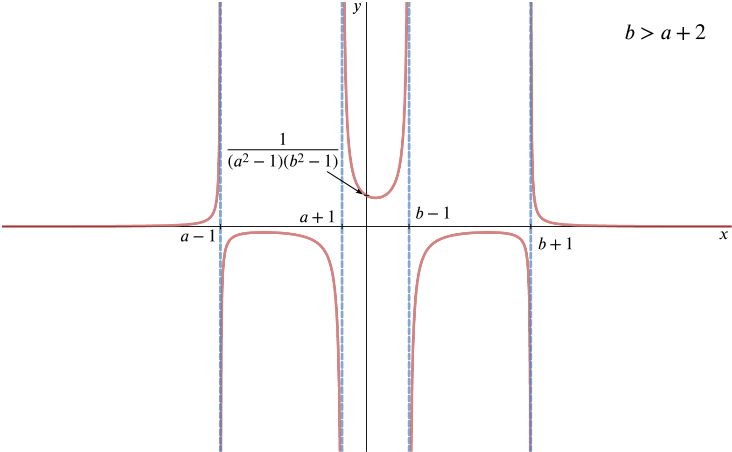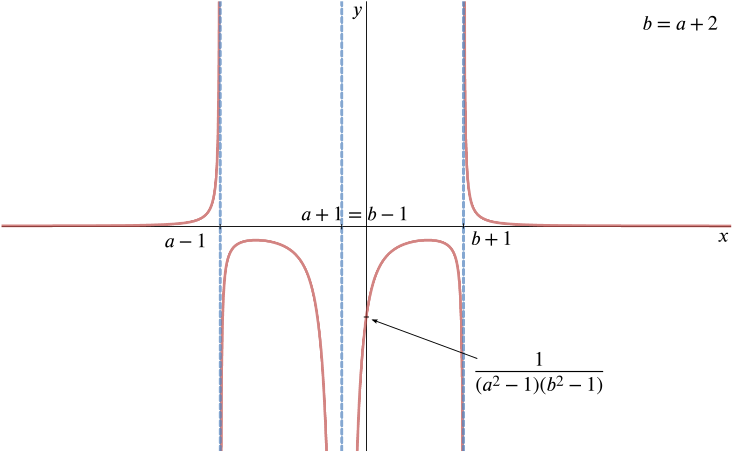Review question

# Can we sketch the curve $y=1/((x-a)^2-1)$? Add to your resource collection Remove from your resource collection Add notes to this resource View your notes for this resource

Ref: R6855

## Solution

1. Sketch the curve $y=f(x)$ where $f(x)=\frac{1}{(x-a)^2-1} \qquad \qquad (x\neq a\pm 1),$ and $a$ is a constant.

Using the difference of two squares, $(x-a)^2 - 1 = ((x-a)-1)(x-a)+1) = (x-(a+1))(x-(a-1))$.

Thus $f(x) = \dfrac{1}{(x-(a+1))(x-(a-1))}$, and we have the vertical asymptotes $x = a-1$ and $x = a+1$.

Near the asymptote $x=a-1$ as $x \rightarrow a-1$ from below $(x-a)^2 \rightarrow 0$ from above so $f(x) \rightarrow \infty$, whereas above $x=a-1, \: \: f(x) \rightarrow -\infty$.

Similarly near the asymptote $x=a+1$ as $x \rightarrow a+1$ from below $(x-a)^2 \rightarrow 0$ from below so $f(x) \rightarrow -\infty$, whereas above $x=a+1, \: \: f(x) \rightarrow \infty$.

As $x \rightarrow \infty, \: \: f(x)$ is positive and tending to zero, and as $x \rightarrow -\infty, \: \: f(x)$ is also positive and tending to zero.

We have the $y$-intercept when $x=0$: $f(0)=\frac{1}{a^2-1}.$

There are no roots to the equation $f(x)=0$, and we can see that the curve will be symmetrical about the line $x=a$.

We can use the symmetry about $x=a$ to find the maximum point of the central branch by evaluating the curve at $x=a$ giving $f(a)=-1$.

We could look for turning points by setting the first derivative equal to zero. To find the first derivative we will need to use quotient rule: $0=f'(x)=-\frac{2(x-a)}{((x-a)^2-1)^2}$ $\iff \quad 2(x-a)=0.$ So the only stationary point is at $x = a$, and $f(a) = -1$.

1. The function $g(x)$ is defined by $g(x)=\frac{1}{((x-a)^2-1)((x-b)^2-1)} \qquad \qquad (x\neq a\pm 1,\,x\neq b \pm 1),$ where $a$ and $b$ are constants, and $b>a$. Sketch the curves $y=g(x)$ in the two cases $b>a+2$ and $b=a+2$, finding the values of $x$ at the stationary points.

We have $g(x) = \dfrac{1}{(x-(a+1))(x-(a-1))(x-(b-1))(x-(b+1))}$, as before.

Thus we have the vertical asymptotes $x = a-1, x = a+1, x = b-1$ and x = $b+1$.

If $b > a+2$, these will be distinct, with $x = b-1$ and x = $b+1$ being to the right of the first two.

As $x \rightarrow \infty, \: \: f(x)$ is positive and tending to zero, and as $x \rightarrow -\infty, \: \: f(x)$ is also positive and tending to zero.

The $y$-intercept is $g(0)=\frac{1}{(a^2-1)(b^2-1)},$ and again there are no roots of $g(x)=0$. This time, the curve will be symmetric about $x=\frac{1}{2}(a+b)$.

Stationary points occur when $g'(x)=0$ so $0=g'(x)=\frac{-2(x-a)}{((x-a)^2-1)^2((x-b)^2-1)}+\frac{-2(x-b)}{((x-a)^2-1)((x-b)^2-1)^2}$ \begin{align*} \iff 0&= (x-a)[(x-b)^2-1]+(x-b)[(x-a)^2-1]\\ &=(x-a)^2(x-b)-(x-b)+(x-a)(x-b)^2-(x-a)\\ &=(x-a)(x-b)\left[(x-a)+(x-b)\right]-\left[(x-a)+(x-b)\right] \\ &=\left((x-a)(x-b)-1\right)(2x-a-b) \end{align*} $\iff [x^2-(a+b)x+(ab-1)](2x-a-b)=0.$ The roots of this are therefore \begin{align*} x&=\frac{1}{2}(a+b)\\ \text{and } x &= \frac{(a+b)\pm\sqrt{(a+b)^2-4(ab-1)}}{2}\\ &=\frac{(a+b)\pm\sqrt{a^2-2ab+b^2+4}}{2}\\ &=\frac{1}{2}(a+b)\pm\frac{1}{2}\sqrt{(a-b)^2+4}. \end{align*}

The discriminant of this is always positive so these two roots are found equally spaced either side of the first root $x=\frac{1}{2}(a+b)$.

We can see that $g\left(\frac{1}{2}(a+b)\right)=\frac{1}{\left(\frac{1}{4}(b-a)^2-1\right)\left(\frac{1}{4}(a-b)^2-1\right)}=\frac{1}{\left(\frac{1}{4}(b-a)^2-1\right)^2}>0.$

When $b>a+2$, we have $b-1>a+1$ so there are four distinct asymptotes and the sketch isGraph of $y=g(x)$ in the case $b>a+2$

When $b=a+2$, we have $b-1=a+1$ so we lose the middle branch and the sketch isGraph of $y=g(x)$ in the case $b=a+2$